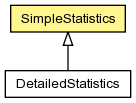## org.jboss.dna.common.statistic Class SimpleStatistics<T extends Number>

```java.lang.Objectorg.jboss.dna.common.statistic.SimpleStatistics<T>
```
Type Parameters:
`T` - the number type used in these statistics
Direct Known Subclasses:
DetailedStatistics

```@ThreadSafe
public class SimpleStatistics<T extends Number>extends Object```Encapsulation of the statistics for a series of values to which new values are frequently added. The statistics include the `minimum`, `maximum`, `total (aggregate sum)`, and `mean (average)`. See `DetailedStatistics` for a subclass that also calculates the `median`, `standard deviation` and the `histogram` of the values.

Constructor Summary
`SimpleStatistics(MathOperations<T> operations)`

Method Summary
` void` `add(T value)`
Add a new value to these statistics.
` int` `getCount()`
Get the number of values that have been measured.
` MathOperations<T>` `getMathOperations()`

` T` `getMaximum()`
Get the maximum value in the series.
` T` `getMean()`
Return the approximate mean (average) value represented as an instance of the operand type.
` double` `getMeanValue()`
Return the mean (average) value.
` T` `getMinimum()`
Get the minimum value in the series.
` T` `getTotal()`
Get the aggregate sum of the values in the series.
` void` `reset()`
Reset the statistics in this object, and clear out any stored information.
` String` `toString()`

Methods inherited from class java.lang.Object
`equals, getClass, hashCode, notify, notifyAll, wait, wait, wait`

Constructor Detail

### SimpleStatistics

`public SimpleStatistics(MathOperations<T> operations)`
Method Detail

`public void add(T value)`
Add a new value to these statistics.

Parameters:
`value` - the new value

### getTotal

`public T getTotal()`
Get the aggregate sum of the values in the series.

Returns:
the total of the values, or 0.0 if the `count` is 0

### getMaximum

`public T getMaximum()`
Get the maximum value in the series.

Returns:
the maximum value, or 0.0 if the `count` is 0

### getMinimum

`public T getMinimum()`
Get the minimum value in the series.

Returns:
the minimum value, or 0.0 if the `count` is 0

### getCount

`public int getCount()`
Get the number of values that have been measured.

Returns:
the count

### getMean

`public T getMean()`
Return the approximate mean (average) value represented as an instance of the operand type. Note that this may truncate if the operand type is not able to have the required precision. For the accurate mean, see `getMeanValue()`.

Returns:
the mean (average), or 0.0 if the `count` is 0

### getMeanValue

`public double getMeanValue()`
Return the mean (average) value.

Returns:
the mean (average), or 0.0 if the `count` is 0
`getMean()`

### reset

`public void reset()`
Reset the statistics in this object, and clear out any stored information.

### getMathOperations

`public MathOperations<T> getMathOperations()`

### toString

`public String toString()`
Overrides:
`toString` in class `Object`